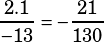Test 8 Section 3 #13

You’re translating from words into math here. Key words to note: “at a constant rate” means you’re dealing with something linear, that has a slope. And “decreased at a constant rate” means you’re looking for a negative slope.

Because the function is a function of t, years are your x-variable and and millions of barrels is your y-variable. Calculate the slope from the numbers the question provides: 4 million barrels in 2000 and 1.9 million barrels in 2013.Note that while no answer choice has that slope in that exact form, choice C is equivalent..

Because all the answer choices have the same y-intercept of 4, you need not think much about it, but it does make sense given the question. There were 4 million barrels in 2000 and t represents years after 2000, so t = 0 when the y-variable is 4.